# How to Calculate Total Work Days or Hours Exclude Weekends/Holidays in Excel

In our daily work we often need to count the total working-days or working-hours for employees then we can pay for them the salary in some cases. But we have to count the total exclude weekends or holidays as we don’t work on these days. So, we need to know how can we calculate the total work days or hours exclude these non-working days. Actually, excel functions are so strong that can be used directly to do calculation for some specific cases, this article will introduce you to calculate total work days/hours by NETWORKDAYS function, now let’s get started.

Before learning the formula, we need to prepare a simple table for demonstration.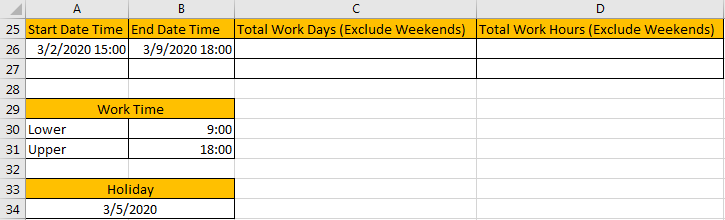In above table, we can see that the normal working day is started from 9:00 (lower) to 18:00 (upper), total 9 hours, and we want to calculate the work days/hours (exclude weekends) between 3/2/2020 15:00 to 3/9/2020 18:00. Holidays is 3/5/2020. Please make sure the format is correct for your date and time. If you are not sure the format for your cells, you can enter Format Cells window to set them by date or time or custom.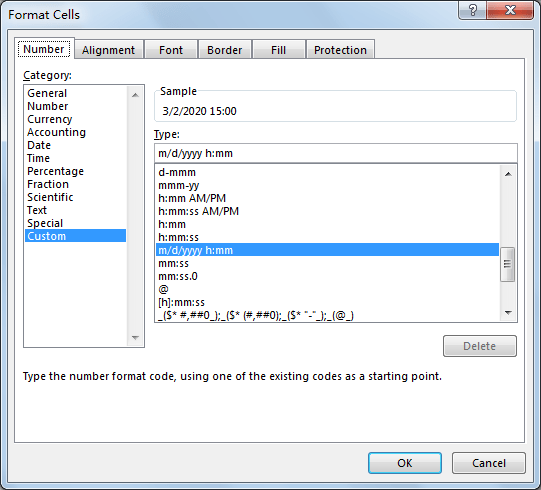## Part 1: Calculate Total Work Days Exclude Weekends

As we mentioned, we will use NETWORKDAYS function to count days. This function is easily to understand, it is used for returning the days between start date and end date, it automatically excludes the weekends.

=NETWORKDAYS(start_date,end_date,holidays)

Step 1: In C26, enter the formula =NETWORKDAYS(A26,B26).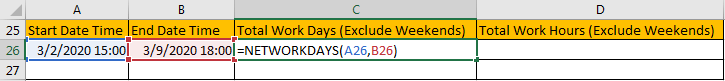Step 2: Click Enter to get result.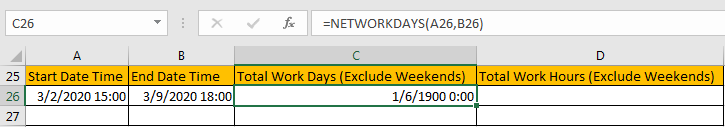Step 3: As we set cell format as date and time, so we need to convert it to general for C26. Select C26, and click dropdown list in Number. Select General.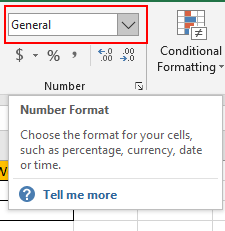Step 4: Verify that 6 is displayed properly. It is an approximate value for total work days, start time and end time are not included into consideration. Anyway, it already excludes the weekends as we expect.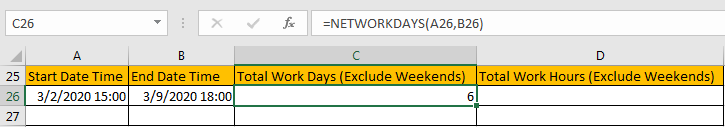## Part 2: Calculate Total Work Days Exclude Holidays

We just need to add holiday parameter into the formula. So, in C26, this time we enter =NETWORKDAYS(A26,B26,A34), then click Enter.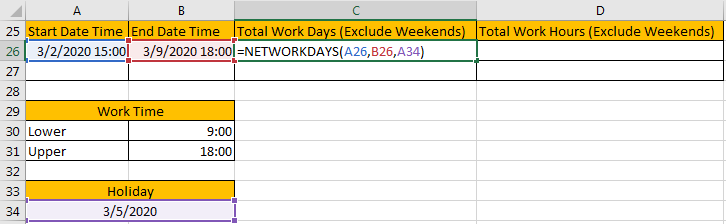Verify that this time 5 is displayed as total work days. Holiday is excluded.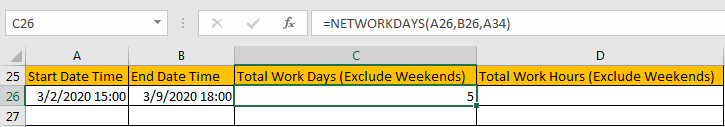## Part 3: Calculate Total Work Hours Exclude Weekends

If we pay for salary based on hours or we need to know an exactly working hours for an employee, we can use NETWORKDAYS function to calculate total work hours as well, but the formula is a little complex, and it also contains some other functions.

Step 1: In D26, enter the formula

=(NETWORKDAYS(A26,B26)-1)*(B31-B30)+IF(NETWORKDAYS(B26,B26),MEDIAN(MOD(B26,1),B31,B30),B31)-MEDIAN(NETWORKDAYS(A26,A26)*MOD(A26,1),B31,B30).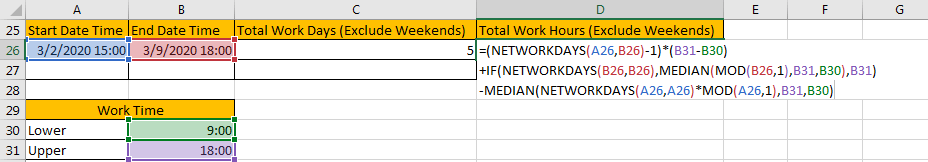You can see details below about formula. I marked parameters in blue.

=(NETWORKDAYS(Start Date Time,End Date Time)-1)*(Upper-Lower)+IF(NETWORKDAYS(End Date Time, End Date Time),MEDIAN(MOD(End Date Time,1),Upper,Lower),Upper)-MEDIAN(NETWORKDAYS(Start Date Time,Start Date Time)*MOD(Start Date Time,1),Upper,Lower)

Step 2: Click Enter to get the result. As currently the format is set as General when calculating total work days in Part 1. So, 2 is displayed in this field now. We need to convert it to proper format later. It also can be displayed as 1/2/1900 0:00 probably if you don’t change format during the whole process.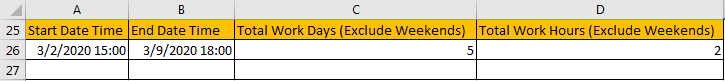Step 3: Select D26, right click to load menu, then select Format Cells to convert format for this cell.Step 4: Under Number tab, select Custom in Category list, then select [h]:mm:ss, click OK. You can also type your own format as well.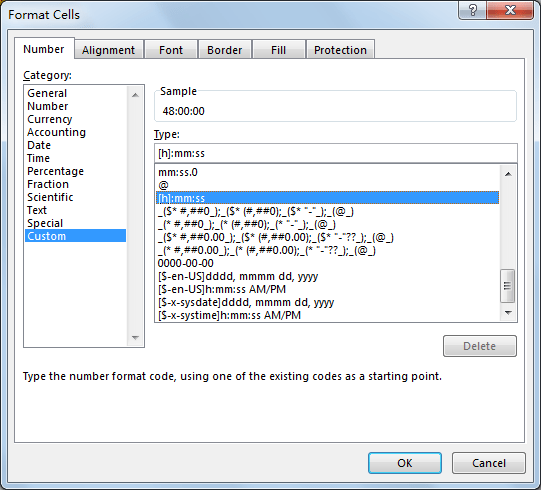Step 5: Verify that 48 hours is calculated properly.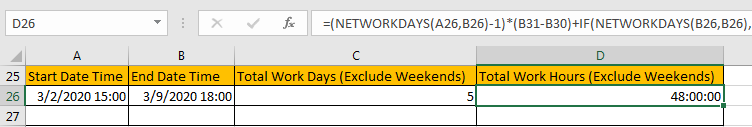Notes:

If the start time and end time are both included between upper and lower period, you can use a simpler formula below:

=(NETWORKDAYS(Start Date Time,End Date Time)-1)*(Upper-Lower)+MOD(End Date Time,1)-MOD(Start Date Time,1)

In this case, enter below formula:

=(NETWORKDAYS(A26,B26)-1)*(B31-B30)+MOD(B26,1)-MOD(A26,1)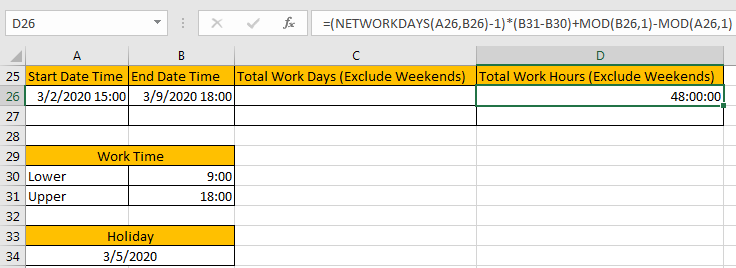## Part 4: Calculate Total Work Hours Exclude Holidays

You can just use 48 to minus 9 hours (Upper-Lower). Or you can also use below format, just add holiday parameter into the first NETWORKDAYS function is ok.

=(NETWORKDAYS(A26,B26,A34)-1)*(B31-B30)+IF(NETWORKDAYS(B26,B26),MEDIAN(MOD(B26,1),B31,B30),B31)-MEDIAN(NETWORKDAYS(A26,A26)*MOD(A26,1),B31,B30)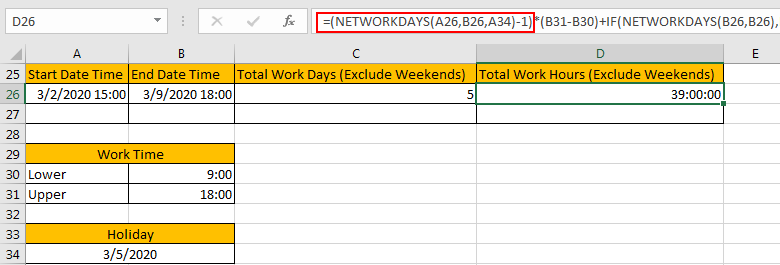### Related Functions

• Excel MEDIAN function
The Excel AVERAGE function returns the median of the given numbers. And the median is the number in the middle of a list of supplied numbers.The syntax of the MEDIAN function is as below:=MEDIAN (number1, [number2], …)….
• Excel IF function
The Excel IF function perform a logical test to return one value if the condition is TRUE and return another value if the condition is FALSE. The IF function is a build-in function in Microsoft Excel and it is categorized as a Logical Function.The syntax of the IF function is as below:= IF (condition, [true_value], [false_value])….
• Excel MOD function
he Excel MOD function returns the remainder of two numbers after division. So you can use the MOD function to get the remainder after a number is divided by a divisor in Excel. The syntax of the MOD function is as below:=MOD (number, divisor)….